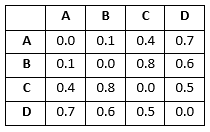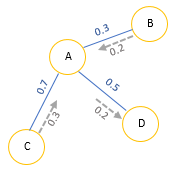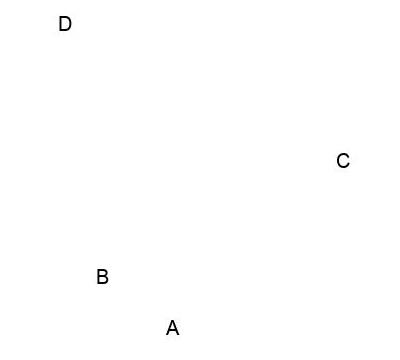Drawing data points in image file using python, Data points in two dimension (2D) based on distance score

 Category: Machine Learning Tags:Viewing data in two dimension (2D) - Code Files

Introduction

Abstracting data reveals many important properties which can’t be seen by analyzing it in record form. Like its always more informative to present summarized graphs of a survey than a tabular data. In this article we will learn how we can draw data points in 2D.

Below we have given adjacency matrix of a graph with four vertices (you can learn about adjacency matrix here), only difference we have given distance between two vertices instead of just a 0/1 flag.Fig 1: Given Distance score in adjacency matrix form

From above table distance between vertex (A, B) = 0.1, (A, C) = 0.4, (B, D) = 0.6 and so on. If we want to draw a graph on paper based on above table would be:

Implementation

Usually in Data Science data will be given in relative form like distance score or similarity score, we won’t be given any coordinates to draw data points. To overcome this situation we have to put random points and rearrange these to satisfy their similarity scores, like for above table data, we know we have to draw four data points and we have distance data between points so we have to put four random points (points which have random coordinates) and keep arranging these until all placed at correct distance from each other.

To arrange data points, we must pick each random point and calculate its distance with all other random data points and based on difference of actual distance (given in table above) and calculated distance, data points should be moved towards or moved away.Fig 3: Randomly placed data points moving in 2D

In above figure, we can see A, B, C and D has placed at random locations and after calculating we figure out B has to be moved towards A by 0.2 so it can satisfy given data where distance between (A, B) = 0.1, same for C which has to be moved towards A by 0.3 and D has to be moved away by 0.2.

Now We will create a method to place random data points and arrange these based on given data:

#number of data points given
n = len(data)

#initializing n random points in 2d
randomPoints = [[random.random(), random.random()] for i in range(n)]
randomDistance = [[0.0 for i in range(n)] for j in range(n)]
lastError = None

for k in range(1000):
#calculating distance between random points
for i in range(n):
for j in range(n):
#distance calculated between random points by √(x1-x2)^2 + (y1-y2)^2
randomDistance[i][j] = math.sqrt(sum([pow(randomPoints[i][x] - randomPoints[j][x], 2) for x in range(len(randomPoints[i]))]))

#how many gradient to move to reduce error, initializing to zero
gradMoveBy = [[0.0, 0.0] for i in range(n)]
totalError = 0.0
#calculating how many gradient to move towards or far, based on given distance and random distance
for i in range(n):
for j in range(n):
if i == j: continue
actualDist = data[i][j] # from given data
randomDist = randomDistance[i][j] # calculated from randomly placed data points
#calculating error percentage in distance (random-actual)/actual
errorPercentage = (randomDist-actualDist)/actualDist
#every point has to be moved away or move towards in propotion to error percentage
gradMoveBy[i] += ((randomPoints[i] - randomPoints[j])/randomDist)*errorPercentage
gradMoveBy[i] += ((randomPoints[i] - randomPoints[j])/randomDist)*errorPercentage
totalError += abs(errorPercentage)

print(totalError)
if lastError and lastError < totalError: break
lastError = totalError

#correcting positions
for i in range(n):

return randomPoints

Above we have a loop up to 1000 which breaks if last error is less then total error, where we calculate total error in every iteration and check if moving data points reduced or increased the error, we keep arranging data points until error is getting reduced, the point it increases we break the loop and that will become final position of random points. Rest of the logic is similar what we have already discussed with illustrations.

Now we will write method to draw these coordinates in JPEG Image

def draw2d(data,labels,jpeg='DataPoints2D.jpg'):
img=Image.new('RGB',(2000,2000),(255,255,255))
draw=ImageDraw.Draw(img)
font = ImageFont.truetype("arial.ttf", 20)
for i in range(len(data)):
x=data[i]*500 #500 times of each point to see it clear in Image
y=data[i]*500
draw.text((x,y),labels[i],(0,0,0),font)
img.save(jpeg,'JPEG')

And now we run above methods:

#given data in array of array
data = [
[0.0, 0.1, 0.4, 0.7],
[0.1, 0.0, 0.8, 0.6],
[0.4, 0.8, 0.0, 0.5],
[0.7, 0.6, 0.5, 0.0]
]
#generated coordinates from random points
pointsOn2d = getCoordinates(data)
print("Created coordinates:") print(pointsOn2d) #creating image file draw2d(pointsOn2d, ['A', 'B', 'C', 'D'])

The output will be printed on console as below as well DataPoints2D.jpg file will be created in directory:

19.810830653013326

17.89922484793863

16.816696082946038

13.282769008418501

10.927259015775212

7.089953362704074

3.4699303193090936

3.438183572381516

3.4549110761492416

Created coordinates:

[[0.4998823452942812, 0.6392305175643206], [0.3589745795332138, 0.53751508621101

77], [0.8397926615825899, 0.304522095212853], [0.282124871482567, 0.030701644666

390825]]

Above output we are printing total error in every iteration and it is reducing from 19.81 to 3.43 and once it is increased to 3.45 we break the loop and print final coordinates, below is the image created by code:Fig 4: Data points displayed in Image file (2D)

Conclusion

There are many packages already exist to display data points in two dimension, above code is given to understand how actually all those packages works or how we can create our own method to display data. We drew A, B, C, D in image file and we can see the similarity between Fig 2 and Fig 4.

 Like0 PeopleNikhil Joshi Ceo & Founder at Dotnetlovers Atricles: 133 Questions: 9 Given Best Solutions: 9 *

Reference:

programming collective intelligence - by Toby Segaran and published by O'Reilly

Login via:x